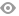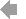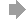﻿ Page 1 - Combined_52_OCR
Basic HTML VersionView Full Version
Page 1 - Combined_52_OCR
P. 1

``````7-4 VIBRATION OF SYSTEMS HAVING DISTRIBUTED MASS AND ELASTICITY
usually results. It can be shown 2 that the frequency that is found by using any shape
except the correct shape always is higher than the actual frequency. Therefore, if more
than one calculation is made, using different assumed shapes, the lowest computed fre­
quency is closest to the actual frequency of the system.                      -ofm-ti::
In many problems for which a classical solution would be possible, the work involved
is excessive. Often a satisfactory answer to such a problem can be obtained by the ap­  ORTH
plication of Rayleigh’s method. In this chapter several examples are worked using both   L
the classical method and Rayleigh’s method. In all, Rayleigh’s method gives a good   system -.
approximation to the correct result with relatively little work. Many other examples of   .-«-h-cted -
solutions to problems by Rayleigh’s method are in the literature.3-4-5        that of ! :
Ritz’s method is a refinement of Rayleigh’s method. A better approximation to tie­  tions as '
fundamental natural frequency can be obtained by its use, and approximations to higher
natural frequencies can be found. In using Ritz’s method, the deflections which are as­
sumed in computing the energies are expressed as functions with one or more undeter­
mined parameters; these parameters are adjusted to make the computed frequencj- a   •a here
minimum. Ritz’s method has been used extensively for the determination of the natural   mode,
frequencies of plates of various shapes, and is discussed in the section on the lateral   For a b
vibrations of plates.                                                         i.c., in th-
Lumped Parameters. A procedure that is useful in many problems for finding ap­
proximations to both the natural frequencies and the mode shapes is to reduce the system
with distributed parameters to one having a finite number of degrees-of-freedom. This
is done by lumping the parameters for each small region into an equivalent mass and
elastic element. Several formalized procedures for doing this and for analyzing the re­  •a here q J
fcth norm;
For a s;
Table 7.2. Approximate Formulas for Natural Frequencies of Systems Having     dimension
Both Concentrated and Distributed Mass

NATURAL
TYPE OF SYSTEM      FREQUENCY       STIFFNESS
LONGITL
circula;
k’m A                                                               Equatio:
D= COIL DIA                       tudinal, t<
d= WIRE DIA                       equations
SPRING WITH MASS ATTACHED            n=NUMBER OF TURNS                 the soluti
cro-vs sec’i
I                                                            In anaiy
k..I. n
tudinal d:
o                                                       stresses in:
D = ROD DIAMETER                 this rnotio:
CIRCULAR ROD, WITH DISC               I = ROD LENGTH                     (onside:
ATTACHED, IN TORSION
by passing
K’. 7.1/A
m/2         m/2
L 48EI
ks“
1=BEAM LENGTH
UNIFORM SIMPLY SUPPORTED
1= MOMENT OF INERTIA
BEAM WITH MASS IN CENTER
E
1=BEAM LENGTH
UNIFORM CANTILEVER BEAM
I = MOMENT OF INERTIA
WITH MASS ON END                                                      * ’r‘ ’-1. (.4``````1   2